Courses

# Liveliness Analysis Notes | EduRev

## Computer Science Engineering (CSE) : Liveliness Analysis Notes | EduRev

The document Liveliness Analysis Notes | EduRev is a part of the Computer Science Engineering (CSE) Course Compiler Design.
All you need of Computer Science Engineering (CSE) at this link: Computer Science Engineering (CSE)

Data-flow Analysis

Idea

Data-flow analysis derives information about the dynamic behavior of a program by only examining the static code
Examples:-

• How many registers do we need for the program on the right?
• Easy bound: the number of variables used (3)
• Better answer is found by considering the dynamic requirements of the program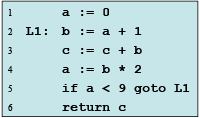Liveness Analysis

➤ Definition:- A variable is live at a particular point in the program if its value at that point will be used in the future (dead, otherwise).
∴ To compute liveness at a given point, we need to look into the future

➤ Motivation: Register Allocation

• A program contains an unbounded number of variables
• Must execute on a machine with a bounded number of registers
• Two variables can use the same register if they are never in use at the same time (i.e, never simultaneously live).
∴ Register allocation uses liveness information

Liveness by Example

➤ What is the live range of b?

• Variable b is read in statement 4, so b is live on the (3 → 4) edge
• Since statement 3 does not assign into b, b is also live on the (2→3) edge
• Statement 2 assigns b, so any value of b on the (1→2) and (5→ 2) edges are not needed, so b is dead along these edges b’s live range is (2→3→4)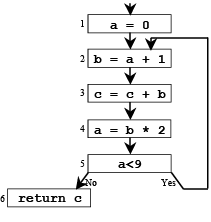Liveness by Example (cont)

• Live range of a – a is live from (1→2) and again from (4→5→2) – a is dead from (2→3→4)
• Live range of b – b is live from (2→3→4)
• Live range of c – c is live from (entry→1→2→3→4→5→2, 5→6)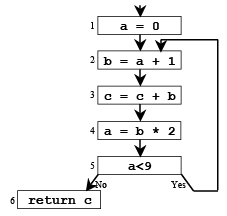Variables a and b are never simultaneously live, so they can share a register

Control Flow Graphs (CFGs)

➤ Definition:- A CFG is a graph whose nodes represent program statements and whose directed edges represent control flow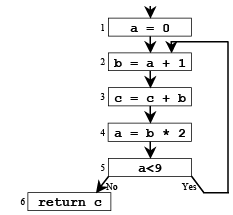Examples
1 a := 0
2 L1: b := a + 1
3 c := c + b
4 a := b * 2
5 if a < 9 goto L1
6 return c

Terminology

➤ Flow Graph Terms

• A CFG node has out-edges that lead to successor nodes and in-edges that come from predecessor nodes
• pred[n] is the set of all predecessors of node n succ[n] is the set of all successors of node n

Examples

• Out-edges of node 5: (5→6) and (5→2)
• succ = {2,6}
• pred = {4}
• pred = {1,5}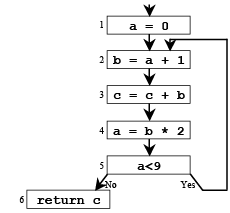Uses and Defs

➤ Def (or definition)

• An assignment of a value to a variable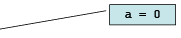• def[v] = set of CFG nodes that define variable v
• def[n] = set of variables that are defined at node n

Use

• A read of a variable’s value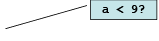• use[v] = set of CFG nodes that use variable v
• use[n] = set of variables that are used at node n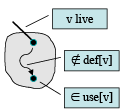More precise definition of liveness

• A variable v is live on a CFG edge if
(1) ∃ a directed path from that edge to a use of v (node in use[v]), and
(2) that path does not go through any def of v (no nodes in def[v])

The Flow of Liveness

Data-flow
Liveness of variables is a property that flows through the edges of the CFG

Direction of Flow

• Liveness flows backwards through the CFG, because the behavior at future nodes determines liveness at a given node
• Consider a
• Consider b
• Later, we’ll see other properties that flow forward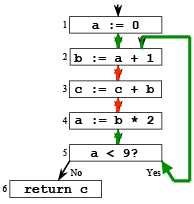Liveness at Nodes

➤ We have liveness on edges- How do we talk about liveness at nodes?

➤ Two More Definitions- A variable is live-out at a node if it is live on any of that node’s outedges

A variable is live-in at a node if it is live on any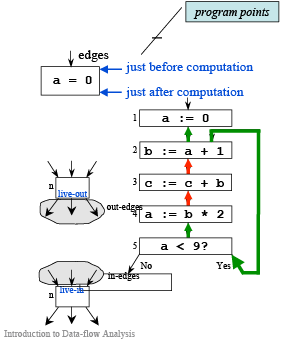Computing Liveness

➤ Rules for computing liveness

(1) Generate liveness: If a variable is in use[n], it is live-in at node n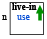(2) Push liveness across edges: If a variable is live-in at a node n then it is live-out at all nodes in pred[n]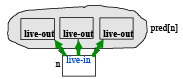(3) Push liveness across nodes: If a variable is live-out at node n and not in def[n] then the variable is also live-in at n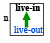➤ Data-flow equations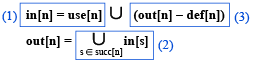Solving the Data-flow Equations

➤ Algorithm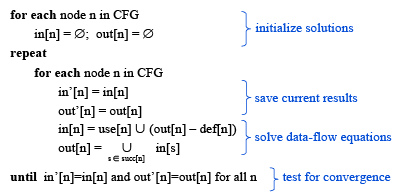This is iterative data-flow analysis (for liveness analysis)

➤ Example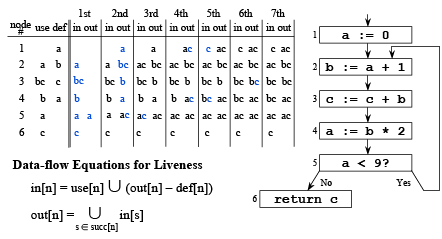➤ Example (cont)

Data-flow Equations for Liveness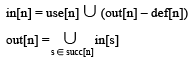➤ Improving Performance

• Consider the (3→4) edge in the graph: out is used to compute in in is used to compute out . . .
• So we should compute the sets in the order: out, in, out, in, . . .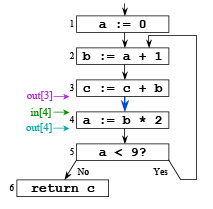The order of computation should follow the direction of flow

Iterating Through the Flow Graph Backwards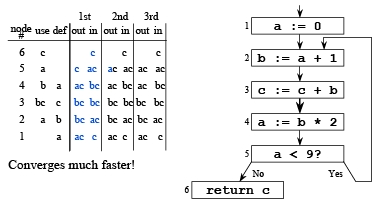Solving the Data-flow Equations (reprise)

➤ Algorithm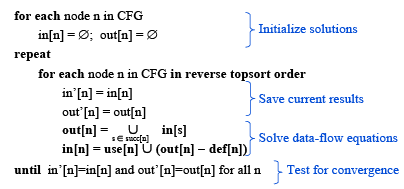Time Complexity

➤ Consider a program of size N

• Has N nodes in the flow graph and at most N variables
• Each live-in or live-out set has at most N elements
• Each set-union operation takes O(N) time
• The for loop body
• constant # of set operations per node
• O(N) nodes ⇒ O(N2) time for the loop
• Each iteration of the repeat loop can only make the set larger
• Each set can contain at most N variables ⇒ 2N2 iterations

Worst case: O(N4)

Typical case: 2 to 3 iterations with good ordering & sparse sets ⇒ O(N) to O(N2)

More Performance Considerations

Basic blocks
Decrease the size of the CFG by merging nodes that have a single predecessor and a single successor into basic blocks

One variable at a time
Instead of computing data-flow information for all variables at once using sets, compute a (simplified) analysis for each variable separately

Representation of sets

• For dense sets, use a bit vector representation
• For sparse sets, use a sorted list (e.g., linked list)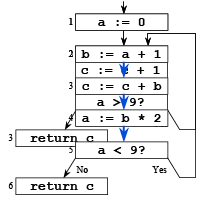Conservative Approximation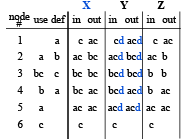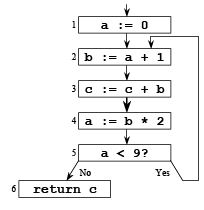➤ Solution X
Our solution as computed on previous slides

Conservative Approximation (cont)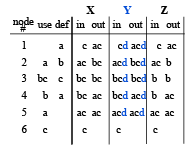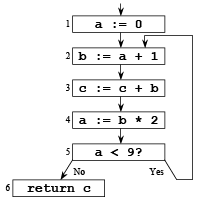➤ Solution Y

• Carries variable d uselessly around the loop
• Does Y solve the equations?
• Is d live?
• Does Y lead to a correct program?

Imprecise conservative solutions ⇒ sub-optimal but correct programs

Conservative Approximation (cont)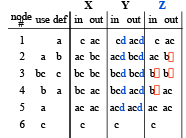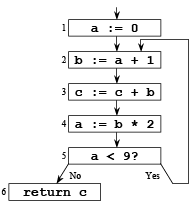➤ Solution Z

• Does not identify c as live in all cases
• Does Z solve the equations?
• Does Z lead to a correct program?

Non-conservative solutions ⇒ incorrect programs

The Need for Approximations

➤ Static vs. Dynamic Liveness

• In the following graph, b*b is always non-negative, so c >= b is always true and a’s value will never be used after node 2

➤ Rule (2) for computing liveness

• Since a is live-in at node 4, it is live out at nodes 3 and 2
• This rule ignores actual control flow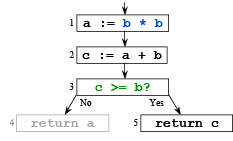No compiler can statically know all a program’s dynamic properties!

Concepts

Liveness

• Use in register allocation
• Generating liveness
• Flow and direction
• Data-flow equations and analysis
• Complexity
• Improving performance (basic blocks, single variable, bit sets)

➤ Control flow graphs

• Predecessors and successors

➤ Defs and uses

➤ Conservative approximation

• Static versus dynamic liveness

Next Time

• Muchnick Ch. 7-7.5

• Other data-flow analyses

➤ Lecture

• Control-flow analysis
• Basic blocks and control-flow graphs
Offer running on EduRev: Apply code STAYHOME200 to get INR 200 off on our premium plan EduRev Infinity!

## Compiler Design

16 videos|44 docs|29 tests

,

,

,

,

,

,

,

,

,

,

,

,

,

,

,

,

,

,

,

,

,

;